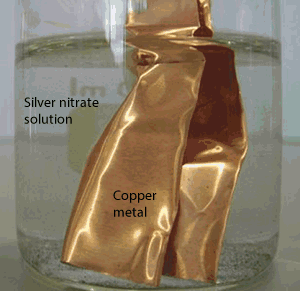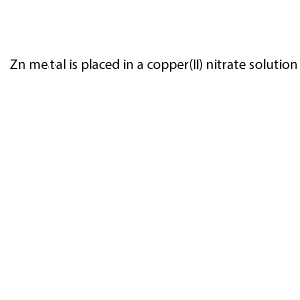Metal displacement reactions in solutions Metal displacement reactions are ones where a metal is replaced by another metal. It is a redox reaction with the general equation given below. A(s) + BD (aq) --> B(s) + AD(aq) In these reactions a more reactive metal displaces a less reactive metal to form a compound. In the above equation solid metal A is more reactive than metal B which is present in a compound. A redox reaction now takes place where solid metal A dissolves and is replaced by solid metal B. A good example is shown on the right when solid copper metal is placed in a solution of silver nitrate. Copper metal displaces the less reactive silver metal and so copper dissolves to form a compound while silver forms a solid metal according to the equation below. Cu(s) + 2AgNO3(aq) --> 2Ag(s) + Cu(NO3)2(aq) This reaction can be used to create some real magic as examplified by the christmas tree reaction. Cu(s) + 2AgNO3(aq) --> 2Ag(s) + Cu(NO3)2(aq)So how do we know if a reaction will occur and how do we write the equation? Example Suppose you are given the following information. "Zinc metal is placed in a solution  of copper(II)nitrate". Is this a metal displacement reaction? If so write the balanced chemical equation. Since metal displacement reactions are redox reactions we can see if a reaction will take place by using the electrochemical series. Step 1 We must first see if Zinc metal, the reductant, is lower than the copper ions (Cu2+). Now the metal, which is the reductant, should react with the strongest oxidant above it in the electrochemical series. Step 2 Once we have verified that a spontaneous reaction will occur we can proceed to write the displacement reaction. Simply switch the position of the metals in the word equation, convert the words to formulae and finally balance the equation.Consider the following. Write the balanced chemical reaction if one is likely to occur. a. Copper metal is placed in a solution of aluminium nitrate    Solution b. Aluminium metal is placed in a solution of nickel(II) nitrate Solution c. Zinc metal is placed in a solution of iron(II) nitrate Solution d. Cobalt metal is placed in a solution of lead(II) nitrate Solution e. Iron metal is placed in a solution of magnesium nitrate Solution